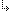- 4.49.1.13. -4. Standard Units4.49. Mouse - Mouse Handling4.49.1. Mouse Unit Procedures and Functions4.49.1.13. SetMouseHandler procedure

## 4.49.1.13. SetMouseHandler procedure

Targets: MS-DOS, Win32 console

Mouse Unit

Installs a Pascal interrupt-driven user's mouse handler (Hnd).

Declaration:
```procedure SetMouseHandler (Mask: DWord;
Buttons,
X, Y,
MovX, MovY: System.Word));```
Remarks:
The Mask parameter defines the classes of the events that call the handler; its format corresponds to the function 0Ch (INT 33h). When Hnd is called the Mask parameter contains the mask with the event type that occurred; Buttons contain the mask of the currently pressed buttons; X and Y contain the cursor's absolute position, and MovX and MovY contain the relative (signed) change of the last cursor position [negative numbers mean left or down; positive mean right or up]. These values are given in mouse position units; to convert to symbols, they need to be divided by 8.

Using SetMouseHandler you can install several handlers with different masks without having to clear previous handlers.

``` bit 0 = mouse movement           (Mask = \$01)
bit 1 = left button pressed      (Mask = \$02)
bit 2 = left button released     (Mask = \$04)
bit 3 = right button pressed     (Mask = \$08)
bit 4 = right button released    (Mask = \$10)
bit 5 = center button pressed    (Mask = \$20)
bit 6 = center button released   (Mask = \$40)
ClearMouseHandler

Example:
```program Test;
{\$ifndef __DOS__}
{\$ifndef __WIN32__}
This program can be compiled for MS-DOS and Win32 console targets only
{\$endif}
{\$endif}
uses
CRT, Mouse;
var
isExit: Boolean := FALSE;
X, Y:   DWord;
star:   Word := \$0F2A;
space:  Word := \$0F00;

procedure MyHnd(Mask, Buttons, X, Y, MovX, MovY: System.Word);
begin
GotoXY(1, 2);
Writeln('XPos = ', MickyToText(X),', YPos = ', MickyToText(Y), '  ');
end;

begin
ClrScr;
Writeln('Press Esc to exit');
HideCursor;
InitMouse;
SetMouseHandler(\$FFFF, MyHnd);
repeat
X := MickyToText(GetMouseX) + 1;
Y := MickyToText(GetMouseY) + 1;
if (LeftButtonPressed) and (Y > 2) and
(Y < 25) then  WriteAttr (X, Y, Star, 1);
if (RightButtonPressed) and (Y > 2) and
(Y < 25) then WriteAttr (X, Y, Space, 1);
if Keypressed then